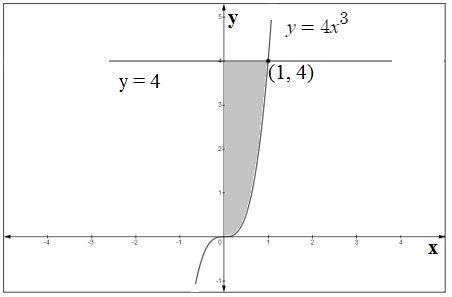# Find the area of the finite region in the first quadrant bounded above by y=4 and below by y=4x^3

Question
99 views

Find the area of the finite region in the first quadrant bounded above by y=4 and below by y=4x^3

check_circle

star
star
star
star
star
1 Rating
Step 1

Given,Step 2

Now, draw the graph and make the common region...

### Want to see the full answer?

See Solution

#### Want to see this answer and more?

Solutions are written by subject experts who are available 24/7. Questions are typically answered within 1 hour.*

See Solution
*Response times may vary by subject and question.
Tagged in